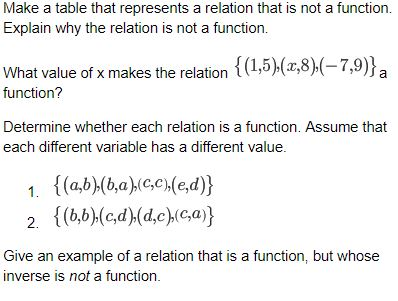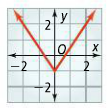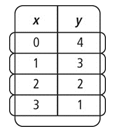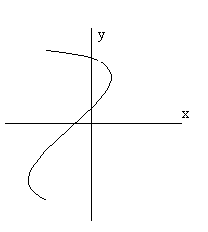#### MAT-HS.F-IF.01

 MAT-HS Targeted Standards(F) Concept: Function(IF) Domain: Interpreting FunctionsCluster: Understand the concept of a function and use function notation. MAT-HS.F-IF.01 Understand that a function from one set (called the domain) to another set (called the range) assigns to each element of the domain exactly one element of the range. If f is a function and x is an element of its domain, then f(x) denotes the output of f corresponding to the input x. The graph of f is the graph of the equation y = f(x).

## Student Learning Targets:

• I can
• I can

• I can
• I can

### Skills (Performance) Targets

• I can use the definition of a function to determine whether a relationship is a function given a table, graph or words.
• I can identify x as an element of the domain and f(x) as an element in the range given the function f.
• I can identify that the graph of the function f is the graph of the function y=f(x).

• I can
• I can

## Proficiency Scale

 Score Description Sample Activity 4.0 (advanced) In addition to Score 3.0, the student demonstrates in-depth inferences and applications regarding more complex material that go beyond end of instruction expectations.3.5 In addition to Score 3.0 performance, the student demonstrates in-depth inferences and applications regarding the more complex content with partial success. 3.0 (proficient) The student can: understand that a function from one set (called the domain) to another set (called the range) assigns to each element of the domain exactly one element of the range. If f is a function and x is an element of its domain, then f(x) denotes the output of f corresponding to the input x. The graph of f is the graph of the equation y = f(x). The student exhibits no major errors or omissions. Is the relation a function (yes or no)? Explain. a.b.Identify the domain and range of the relation. Is this relation a function (yes or no)?  Explain. (-2, 0.5), ( 0,2.5), (4,6.5), (5,2.5) Graph f (x) = 2x + 2 2.5 The student demonstrates no major errors or omissions regarding the simpler details and processes (Score 2.0 content) and partial knowledge of the more complex ideas and processes (Score 3.0 content). 2.0 (progressing) There are no major errors or omissions regarding the simpler details and processes as the student: Recognize and recall basic vocabulary such as: range domain relation function notation vertical line test However, the student exhibits major errors or omissions regarding the more complex ideas and processes. Find the range of the relation.(4, -2), (-2, 3), (1, -3). Find the domain of the relation. (2, 4), (8, 11), (9, 1), (4, 2) Does the relation pass a vertical line test?Identify the domain and range for the given set of ordered pairs.  Is this relation a function?(7,2), (-1,4), (2,-2), (-6,5), (0,0) 1.5 The student demonstrates partial knowledge of the simpler details and processes (Score 2.0 content) but exhibits major errors or omissions regarding the more complex ideas and procedures (Score 3.0 content). 1.0 (beginning) With help, the student demonstrates a partial understanding of some of the simpler details and processes (Score 2.0 content) and some of the more complex ideas and processes (Score 3.0 content). - 0.5 With help, the student demonstrates a partial understanding of some of the simpler details and processes (Score 2.0 content) but not the more complex ideas and processes (Score 3.0 content). 0.0 Even with help, the student demonstrates no understanding or skill. -

## Resources

 Web Vocab function domain range